Next: Perihelion precession of Mercury Up: Orbits in central force Previous: Motion in nearly circular

# Perihelion precession of planets

The solar system consists of eight major planets (Mercury to Neptune) moving around the Sun in slightly elliptical orbits that are approximately coplanar with one another. According to Chapter 4, if we neglect the relatively weak interplanetary gravitational interactions, the perihelia of the various planets (i.e., the points on their orbits at which they are closest to the Sun) remain fixed in space. However, once these interactions are taken into account, it turns out that the planetary perihelia all slowly precess in a prograde manner (i.e., rotate in the same direction as the orbital motion) around the Sun.5.1 We can calculate the approximate rate of perihelion precession of a given planet by treating the other planets as uniform concentric rings, centered on the Sun, of mass equal to the planetary mass, and radius equal to the mean orbital radius. This method of calculation, which is due to Gauss, is equivalent to averaging the interplanetary gravitational interactions over the orbits of the other planets. It is reasonable to do this because the timescale associated with the perihelion precession is very much longer than the orbital period of any planet in the solar system. Thus, by treating the other planets as rings, we can calculate the mean gravitational perturbation due to these planets, and, thereby, determine the desired precession rate. (Actually, Gauss also incorporated the eccentricities, and non-uniform angular velocities, of the planetary orbits into his original calculation.)

We can conveniently index the planets in the solar system by designating Mercury as planet 1, and Neptune as planet 8. Let the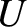and the, for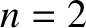, be the planetary masses and mean orbital radii, respectively. Furthermore, let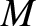be the mass of the Sun. It follows, from Section 3.7, that the gravitational potential generated in the vicinity of the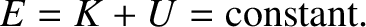th planet by the Sun and the other planets is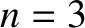(5.21)

where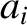. Here,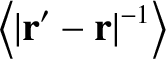is a Legendre polynomial (Abramowitz and Stegun 1965b). The radial force per unit mass acting on theth planet is written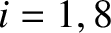. Hence, it is easily demonstrated that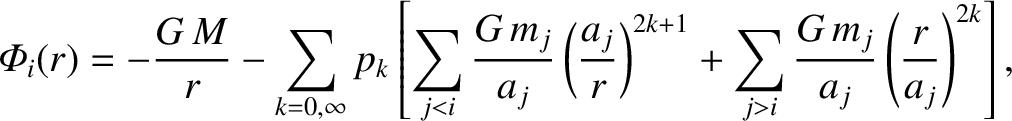(5.22)

to first order in the ratio of the planetary masses to the solar mass. Thus, according to Equation (5.19), the apsidal angle for theth planet is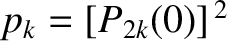(5.23)

Hence, the perihelion of theth planet advances by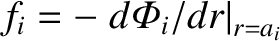(5.24)

radians per revolution around the Sun. Of course, the time required for a single revolution is the orbital period,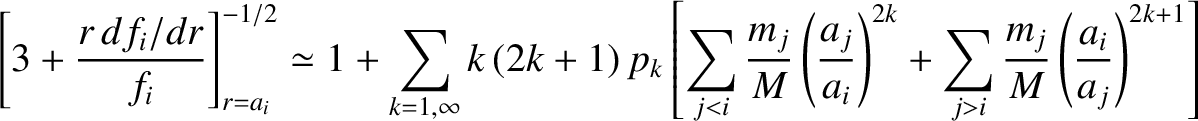. Thus, the rate of perihelion precession, in arc seconds per year, is given by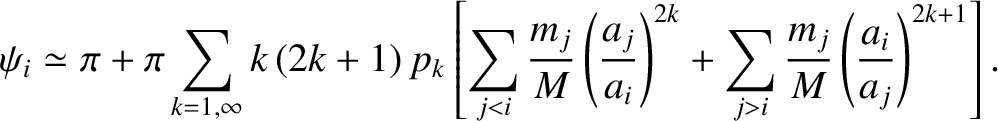(5.25)

12pt

Table: Observed and theoretical planetary perihelion precession rates (at J2000). Source: Standish and Williams (1992).
 Planet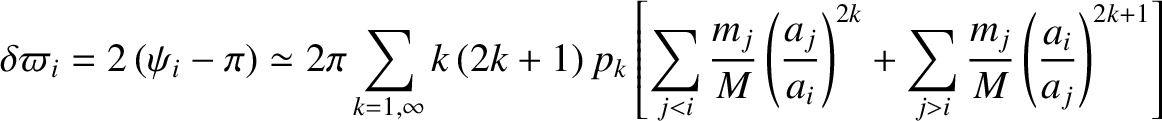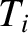Mercury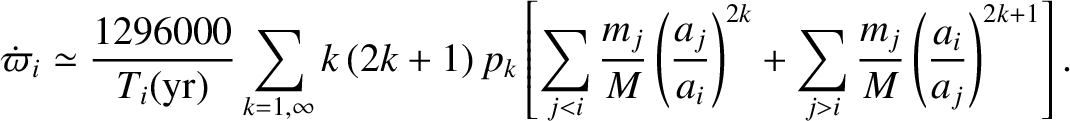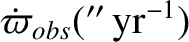Venus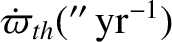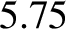Earth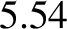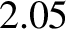Mars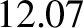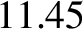Jupiter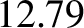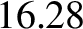Saturn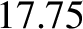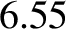Uranus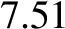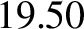Neptune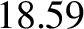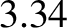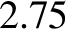Table 5.1 and Figure 5.1 compare the observed perihelion precession rates of the planets with the theoretical rates calculated from Equation (5.25) and the planetary data given in Table 4.1. It can be seen that there is excellent agreement between the two, except for the planet Venus. The main reason for this is that Venus has an unusually low eccentricity (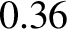), which renders its perihelion point extremely sensitive to small perturbations.Next: Perihelion precession of Mercury Up: Orbits in central force Previous: Motion in nearly circular
Richard Fitzpatrick 2016-03-31